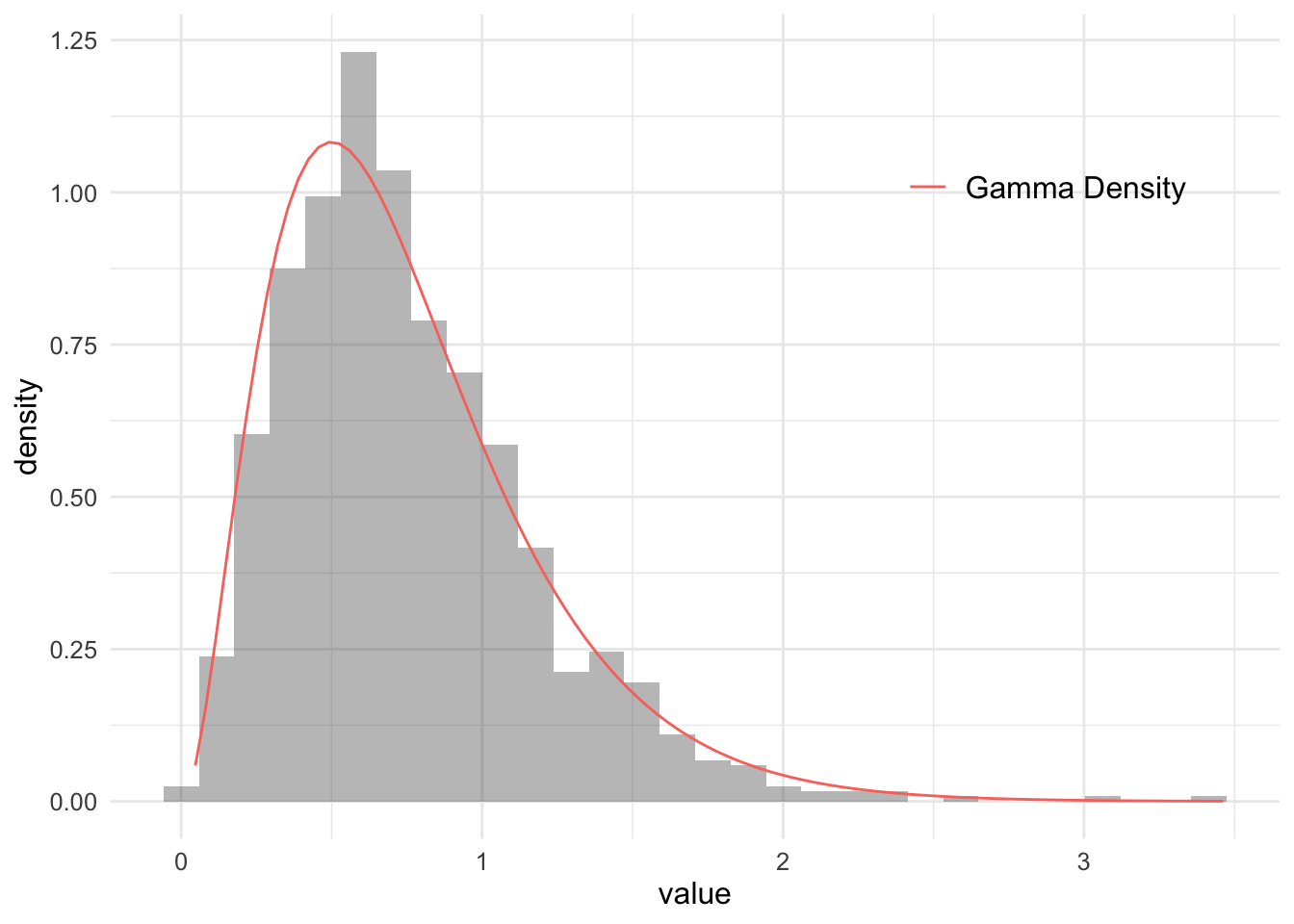# Sampling from a distribution with a known CDF

R
Author

Jonny Law

Published

February 25, 2019

A distribution with an inverse cumulative distribution function (CDF) can be sampled from using just samples from $$U[0, 1]$$. The inverse CDF (sometimes called the quantile function) is the value of $$x$$ such that $$F_X(x) = Pr(X \leq x) = p$$. Consider a that a transformation $$g: [0, 1] \rightarrow \mathbb{R}$$, exists which takes a value sampled from the standard uniform distribution $$u \sim U[0, 1]$$ and returns a value distributed according to the target distribution. Then the inverse CDF can be written as:

$Pr(g(U) \leq x) = Pr(U \leq g^{-1}(x)) = g^{-1}(x)$

Since the CDF of the uniform distribution over the interval $$[0, 1]$$ is:

\begin{align*} F_U(u) = \begin{cases} 0 & u < 0 \\ u & u \in [0, 1) \\ 1 & u \geq 1 \end{cases} \end{align*}

Then $$F_x^{-1}(X) = g(x)$$ as required. The algorithm below summarises the inverse sampling procedure.

1. Sample $$u \sim U[0, 1]$$
2. Evaluate $$x = F^{-1}(u)$$
3. Return $$x$$

Most statistical packages will expose the quantile function for common distributions making it practical to use inverse sampling. The figure below shows a histogram of 1,000 simulated values from a $$\textrm{Gamma}(3, 4)$$ distribution using the inverse CDF method, the analytical density is plotted in red.

The figure below shows samples from $$\textrm{Gamma}(3, 4)$$ using the inverse CDF method plotted with the analytical PDF.

inverse_cdf_sample <- function(inv_cdf) {
u <- runif(1)
inv_cdf(u)
}

inv_cdf <- function(x) qgamma(p = x, shape = 3, rate = 4)
gamma_samples <- replicate(1000, inverse_cdf_sample(inv_cdf))

ggplot(tibble(gamma_samples)) +
geom_histogram(aes(x = gamma_samples, y = ..density..), alpha = 0.4) +
stat_function(
fun = function(x) dgamma(x, shape = 3, rate = 4),
aes(colour = "Gamma Density")
) +
theme(
text = element_text(size = 12), legend.title = element_blank(),
legend.text = element_text(size = rel(1.0)), legend.position = c(0.8, 0.8)
) +
ylab("density") +
xlab("value")
stat_bin() using bins = 30. Pick better value with binwidth.## Citation

BibTeX citation:
@online{law2019,
author = {Jonny Law},
title = {Sampling from a Distribution with a Known {CDF}},
date = {2019-02-25},
langid = {en}
}

For attribution, please cite this work as:
Jonny Law. 2019. “Sampling from a Distribution with a Known CDF.” February 25, 2019.